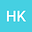•Instrumentally measurable earthquake precursors are derived by considering earthquakes as a phase transition in cellular automaton. The existence of the phase transition in CA-184 is implied by considering the space-time diagram of the CA-184 as the worldsheet of the Polyakov action in string theory. The CA-184 is the rule-184 cellular automaton (CA), which is a special case of Burgers cellular automaton (BCA) rigorously derived through transforming the one-dimensional Burgers equation. Then, $p$-CA-184, the CA-184 with probabilistic fluctuation and $p$-BCA, the BCA with probabilistic fluctuation, are associat-ed with the earthquake. The Fourier transforms of $p$-CA-184 and $p$-BCA dynamics near the phase transition reproduce the Fourier transforms of the ground vibration data before and after the earthquake, respectively. Consequently, we consider an earthquake as the phase transition of CA-184. Two precursors of the phase transition of the CA-184, therefore the earthquake precursors, are derived with $p$-CA-184 by introducing the Gumbel distribution defined in the framework of extreme value theory. To evaluate the pre-cursors, the ground vibration data measured at three locations over a period of approxi-mately 10 years has been investigated. One of the derived precursors is observed before every studied earthquake with seismic intensity greater than 4, and the other precursor is observed selectively before the large earthquake of magnitude 9. Furthermore, the two pre-cursors calculated for different frequencies and time scales are observed at similar timing before the magnitude 9 earthquake. The phase transition model of earthquakes provides the practical and reliable earthquake prediction method.